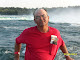# /* // Set the date we're counting down to var countDownDate = new Date("Jan 1, 2019 00:00:00").getTime(); // Update the count down every 1 second var x = setInterval(function() { // Get todays date and time var now = new Date().getTime(); // Find the distance between now and the count down date var distance = countDownDate - now; // Time calculations for days, hours, minutes and seconds var days = Math.floor(distance / (1000 * 60 * 60 * 24)); var hours = Math.floor((distance % (1000 * 60 * 60 * 24)) / (1000 * 60 * 60)); var minutes = Math.floor((distance % (1000 * 60 * 60)) / (1000 * 60)); var seconds = Math.floor((distance % (1000 * 60)) / 1000); // Display the result in the element with id="newyear" document.getElementById("newyear").innerHTML = "There are only " + days + " days until the new year begins!"; //+ hours + "h " + minutes + "m " + seconds + "s "; // If the count down is finished, write some text if (distance < 0) { clearInterval(x); document.getElementById("newyear").innerHTML = "Happy New Year!"; } }, 1000);*/

## This does not rquire a candy thermometer and looks like it will be fairly easy to make...

#### This Is The Only Fudge Recipe You Will Need This Holiday Season

Fudge is an excellent dessert to whip up during the holiday season, and considering this was reportedly one of former first lady Mamie Eisenhower's go-to recipes - it's practically as classic as apple pie. In other words: You'll probably want to make room for this one on your holiday dessert